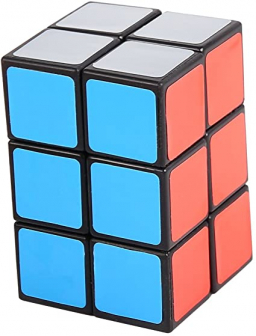# Lengths 63174

The block has a square base of 36 dm2, and its height is 1/3 of the length of the base edge. Find the sum of the lengths of all edges of a block.

x =  56 dm

### Step-by-step explanation:Did you find an error or inaccuracy? Feel free to write us. Thank you!

Tips for related online calculators
Are you looking for help with calculating roots of a quadratic equation?
Need help calculating sum, simplifying, or multiplying fractions? Try our fraction calculator.
Do you want to convert length units?

#### Grade of the word problem:

We encourage you to watch this tutorial video on this math problem: